In both physical and organic chemistry, it’s important to understand the state of atoms. We learn how atoms bond with each other to form molecules. Knowing the state of these bonds allows us to understand how the synthetic reactions of organic compounds proceed.

The bonding of molecules is known as HOMO and LUMO. These are also called bonding orbital and anti-bonding orbital: the HOMO is involved in bonding orbitals, and the LUMO is involved in anti-bonding orbitals.

In physics, HOMO and LUMO are the fields of quantum mechanics. However, there are many difficult formulas in physics textbooks that are difficult to understand.

If you just want to understand the concept of bonding, you don’t need the formulas. Bonding orbitals and anti-bonding orbitals tend to be abstract concepts, but we will try to explain them as simply and clearly as possible.

## Frontier Orbital Theory of Quantum Mechanics with Wave Functions

Quantum mechanics is the study of small matter, such as electrons, to understand it. In quantum mechanics, there is something called a frontier orbital theory. In simple terms, the following is the frontier startup theory.

• Chemical reactions involve HOMO and LUMO.

In quantum mechanics, it is represented by a wave function. Electrons are a type of wave, and we use the wave function.

As you can see from the waves in the ocean, the waves ripple up and down. When waves of the same phase are combined, the waves become larger because they overlap with each other. On the other hand, when waves of opposite phase are combined, they cancel each other out, and no vibration occurs.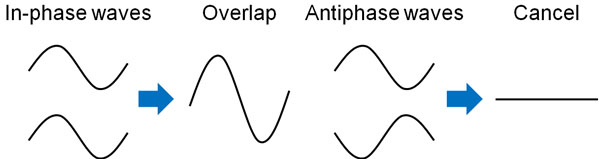The term “wave function” can be difficult for us to understand. Rather, we can understand that electrons are a type of wave.

### Difference Between Bonding and Anti-bonding Orbitals

Once we understand that electrons have properties as waves and that they enhance or cancel each ‘s properties, we can understand the concept of HOMO and LUMO.

HOMO stands for Highest Occupied Molecular Orbital, and HOMO refers to a bonding orbital. On the other hand, LUMO stands for Lowest Unoccupied Molecular Orbital, and LUMO refers to anti-bonding orbitals.

What is the difference between a bonding orbital and an anti-bonding orbital? It is literally the bonding orbitals that are involved in the bonding of the molecule. Also, it is the anti-bonding orbitals that are involved in the cleavage of the molecular bond.

First, let’s consider the example of the hydrogen molecule. A hydrogen atom has a single electron and an s-orbital. When two hydrogen atoms of the in-phase come close to each other, the two hydrogen atoms will overlap. In other words, the atoms bond with each other to form a molecule.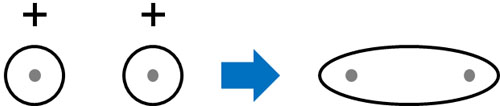Atoms have protons. Therefore, atoms repel each other. However, since electrons have a negative charge, the presence of electrons between hydrogen atoms creates a stable state and allows them to form bonds with each other. The electrons help the hydrogen molecules to form bonds.

This is a bonding orbital. The bonding orbitals (HOMO) are created by combining orbitals that are in phase. The result is the creation of bonds between atoms.

### The Anti-Bonding Orbitals (LUMO) Have Nodes and High Energy

In contrast, what about the anti-phase wave? In the case of anti-phase, the situation is different from the previous one. Because of the anti-phase, the waves in the middle of the hydrogen atoms are completely zero. This is because they cancel each other’s waves due to the anti-phase.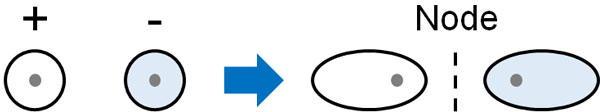The middle part where the wave does not exist is called a node. In the node, the probability of the existence of electrons is zero. Even if there are electrons in the orbitals, there is no electron density between the two hydrogen atoms. Therefore, it does not help bond formation, but rather works to cleave the bond. This is called an anti-bonding orbital (LUMO).

When comparing the HOMO and LUMO orbitals, the HOMO orbitals are naturally lower in energy than the LUMO. Hydrogen atoms share electrons with each other and form molecules of hydrogen to achieve lower energy.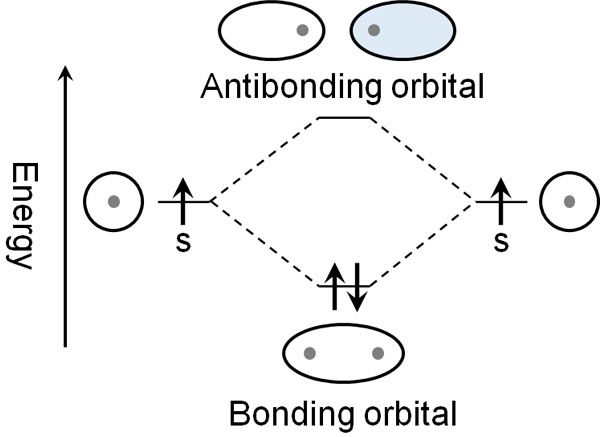In contrast, the anti-bonding orbitals have higher energy. The LUMO is a higher energy state than the s-orbital of the hydrogen atom.

When an organic compound reacts, the bond must be broken. By giving energy to the molecule, if the electronic state is led to an anti-bonding orbital, the bond between the molecules can be broken. Then an organic chemical reaction occurs, and the molecules form new bonds with each other.

The energy difference between the HOMO and LUMO is called the HOMO-LUMO gap. By providing the energy equivalent of the energy gap from the outside, the electron moves into anti-bonding orbitals, resulting in an unstable state.

For example, in the case of a bromine molecule (Br2), the bond is broken by exposing it to light energy. This is because, as a result of the addition of the light energy corresponding to the HOMO-LUMO gap, the electron state becomes an anti-bonding orbital, which breaks the bond and becomes a bromine atom.

### The Reason Why Helium Doesn’t Become a Molecule Has to Do with Its Anti-Bonding Orbital

Once you learn about bonding and anti-bonding orbitals, you will understand why helium exists as an atom rather than a molecule.

While a hydrogen atom has one electron, helium has two electrons. What if helium (He) forms a molecule and becomes He2? In this case, two electrons would be in the bonding orbital, and at the same time, two electrons would be in the anti-bonding orbital.

The situation is as follows.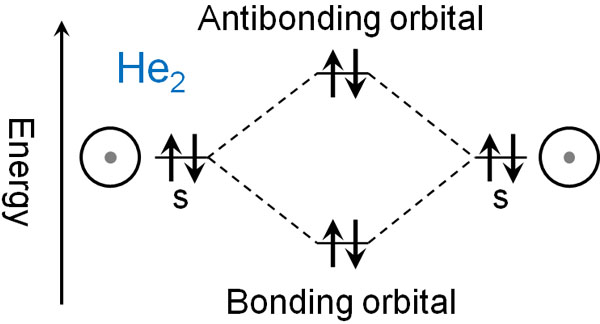When electrons enter only the bonding orbitals, the molecule is stable and bonds are formed, as in hydrogen. In helium, however, the electrons enter into the anti-bonding orbital.

The anti-bonding orbitals act as a bond cleaver. So even if a helium molecule is formed, the bond is immediately cleaved, and the molecule returns to a helium atom. This is the reason why helium does not form bonds and exists as an atom.

## HOMO and LUMO by σ and σ* Bonds in p Orbitals

In the explanation so far, we have used the s-orbital of hydrogen atoms. However, atoms other than hydrogen atoms have not only s-orbital but also p-orbitals. There are also several types of covalent bonds with electron orbitals, such as σ-orbitals and π-orbitals.

How should we think about these orbitals and bonds? Next, let’s consider not only s orbitals but also p orbitals.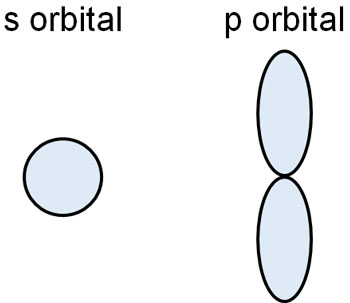Atoms such as carbon, nitrogen and oxygen have more electrons. As a result, they have p orbitals as well as s orbitals.

When molecules are connected to each other, they always form σ-bond (sigma bond) at first. Each atom puts out electrons to each other to form a single bond. When the p orbitals make a bond, the waves will have different properties, just like the s orbitals. In the same phase, they strengthen each other, creating a strong covalent bond. This is the σ bond.

In contrast, in the anti-phase, there is a point in the middle where the electron density becomes zero. This is an anti-bonding orbital, which creates a node.

The shape of the electron cloud is different between s orbitals and p orbitals. However, similarly, the differences of in-phase and anti-phase create HOMO and LUMO. In the sigma bond of p orbitals, the following image is used to form bonding and anti-bonding orbitals.In the case of bonding orbitals, all single bonds are σ-bonds. On the other hand, for anti-bonding orbitals, they are called σ* bonds. Although they are all sigma bonds, if they are involved in the cleavage of the bond in the LUMO, it is a σ* bond.

With a σ bond, the bond energy is very strong. It is a strong bond, but when you add energy to it, and it becomes a σ* bond, which is an anti-bonding orbital, the single bond becomes cleavable.

### π Bonds and π* Bonds When Making Double and Triple Bonds with p Bonds

In the bonding of p orbitals, there are not only σ bonds (sigma bonds) but also π bonds (pi bonds). A single bond results in a σ bond, while a π bond is formed when making a double or triple bond. There are two types of covalent bonds: σ bonds and π bonds.

In a π bond, what do HOMO and LUMO look like? In a π bond, the electrons do not directly overlap; the p orbitals extend up and down, and form a bond by thinly overlapping each other. In the in-phase case, the bond formed by this process is a π bond.

Any single bond is a sigma bond, but when the number of bonds increases, such as a double bond, the new bond is a π bond.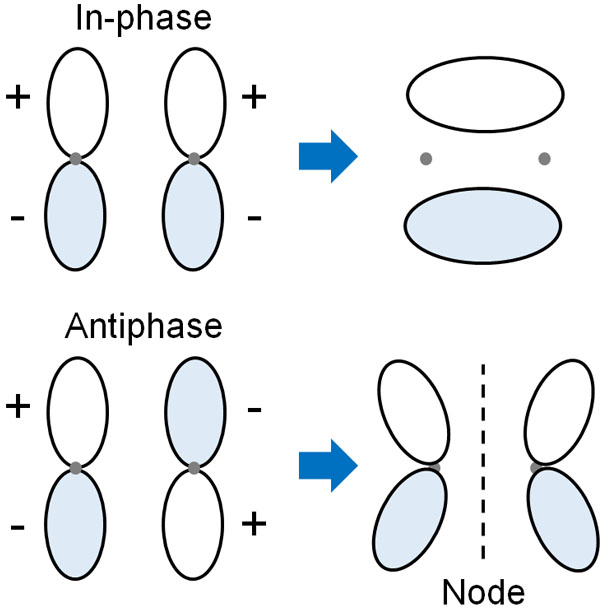In contrast, in the anti-phase, the orbitals are anti-bonding. They repel each other, and the electron density is zero at the midpoint of the pi orbital. The creation of a node causes the bond to break. The π bond in an anti-bonding orbital is a π* orbital.

## HOMO and LUMO at Butadiene

Are the properties of the HOMO and LUMO different from those of the bonding and anti-bonding orbitals? One of the bonding orbitals is HOMO. And one of the anti-bonding orbitals is LUMO. Let’s understand that there are several bonding orbitals and anti-bonding orbitals, and one of them is HOMO and LUMO.

In explaining HOMO and LUMO, 1,3-butadiene is frequently used as an example; 1,3-butadiene has two double bonds, and here is the structure of butadiene.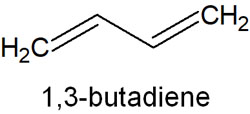If a molecule has a double bond, it has a pi-bond; one pi-bond produces one bonding orbital and one anti-bonding orbital.

Butadiene has two pi bonds (double bonds). These are conjugated structures, meaning that they have two bonding and two anti-bonding orbitals each. The more nodes there are, the more unstable the compound becomes. Therefore, arranged in energy order, butadiene can be divided into the following orbitals.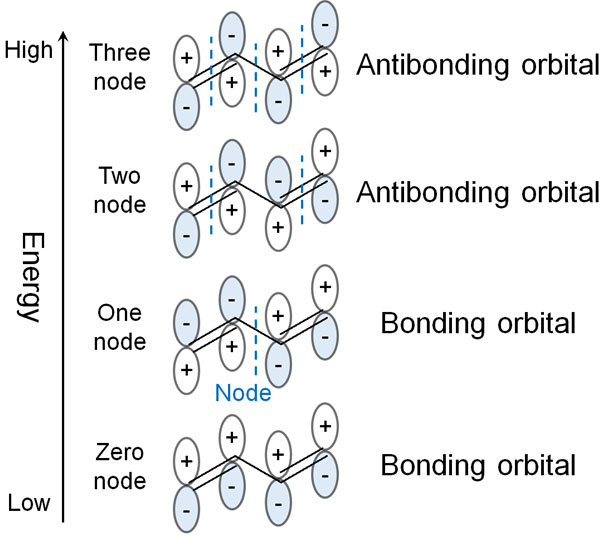One pi bond has two electrons, because one p-orbital can contain two electrons.

Butadiene has two π bonds in it. So the two π bonds in butadiene have four electrons. If we put the electrons in energy order, we get the following.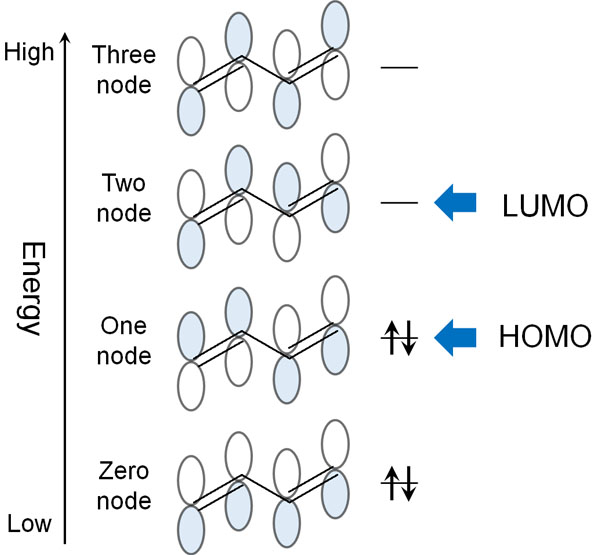The orbitals that contain electrons are bonding orbitals. The orbit with the highest energy among these bonding orbitals is the HOMO. This is the meaning of the Highest Occupied Molecular Orbital (HOMO).

On the other hand, the orbital without any electrons is anti-bonding orbitals. Among these anti-bonding orbitals, the lowest energy orbital is LUMO. This is the meaning of Lowest Unoccupied Molecular Orbital (LUMO).

### Conjugate Structure Changes the Energy and Spectrum of the HOMO-LUMO Gap

Butadiene has two double bonds. So what happens when the number of these conjugated structures increases? In this case, the energy gap between the HOMO and the LUMO becomes smaller.

If there is only one double bond, there will be one bonding orbital and one anti-bonding orbital. However, in the case of butadiene with a conjugated structure, there are two bonding and two anti-bonding orbitals. What happens if the number of double bonds increases further and the conjugated structure extends? As a result, the number of bonding and anti-bonding orbitals increases.

As the number of conjugated structures increases, the HOMO-LUMO gap becomes correspondingly smaller.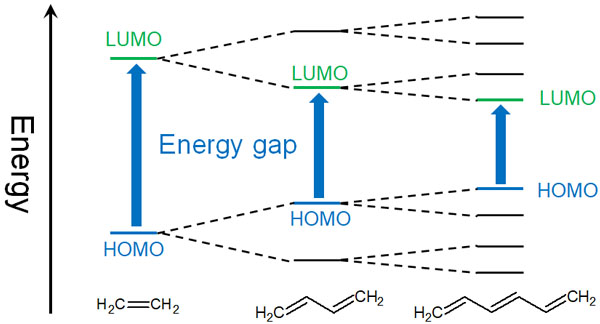In compounds with a conjugated structure, the color is easily absorbed. As the conjugated structure increases, the energy difference between the HOMO and the LUMO becomes smaller, and the electrons become unstable with just a small amount of energy applied.

It is known that as the conjugated structure increases, the absorption spectrum (the wavelength at which light is absorbed) shifts to longer wavelengths when the compound is dissolved in solution. The longer the wavelength, the weaker the energy of the light. Therefore, the following occurs

1. Conjugated structure increases.
2. The HOMO-LUMO gap gets smaller.
3. Even a slight addition of energy makes it unstable.
4. Absorb long-wavelength (low energy) light.

As the conjugation increases, the HOMO becomes higher and the LUMO becomes lower. As a result, it is easier to absorb long-wavelength (low energy) light.

What happens if the difference between HOMO and LUMO disappears? In this case, it becomes like a metal. In metals, there is no energy difference between the HOMO and the LUMO, and electrons can move freely through the metal.

## There Are Different Types of Electron Orbitals in Covalent Bonds

When atoms are bonded together, they become molecules. These molecules contain electrons, and electrons are able to move. However, electrons are not free to move, and they move according to rules.

Bonding orbitals and anti-bonding orbitals are tools for understanding the state of electrons and their bonding. In addition, there are HOMO and LUMO orbitals among the bonding and anti-bonding orbitals. In the wave function of quantum mechanics, we treat electrons as waves. Then we can understand the difference between HOMO and LUMO.

When you study physical and organic chemistry, one of the first things you learn about is bonding and anti-bonding orbitals. However, it is difficult to understand the difference between them, what they mean and how to distinguish between them and their energies.

Even so, once you recognize that electrons are waves and understand that they overlap and cancel each other, you will understand the concepts of HOMO and LUMO. Knowing the state of the electrons is important for learning how compounds react. Make sure you understand what the electron orbitals are at the beginning.